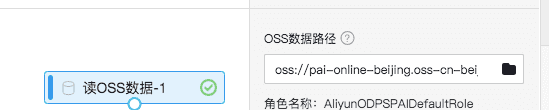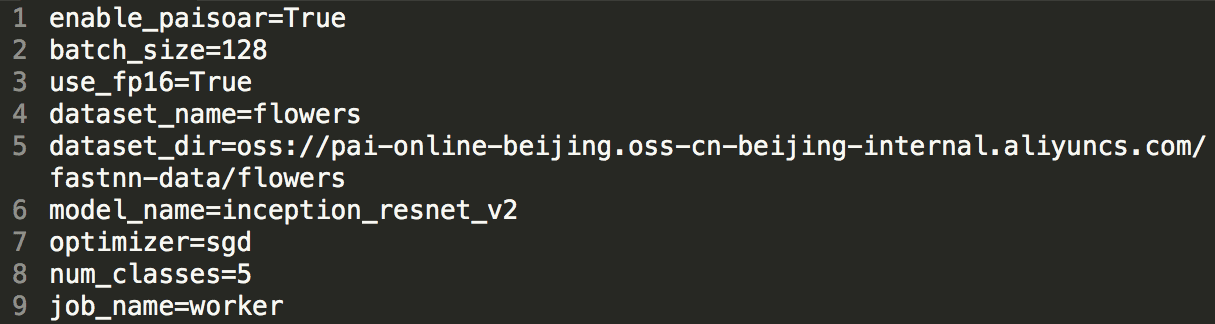Search
Document Center

# Use the FastNN repository

Last Updated: May 14, 2020

Machine Learning Platform for AI (PAI) provides Fast Neural Networks (FastNN), which is a distributed neural network repository based on the PAISoar framework. Currently, FastNN supports classic algorithms such as Inception, Resnet, and VGG. More advanced models will be available in the future. FastNN is built into PAI Studio. To try it out, log on to PAI Studio and create an experiment by clicking the corresponding template on the homepage.

# Custom development method

## 1. Data source preparation

To facilitate trying FastNN in PAI, we have downloaded and converted the cifar10, mnist, and flowers data to tfrecord data and stored the converted data in the open Object Storage Service (OSS). The data can be accessed through the Read File Data or OSS Data Synchronization components of PAI. The following table lists the storage paths in OSS.

Dataset Number of classes Training set Test set Storage path
mnist 10 3320 350 China (Beijing): oss://pai-online-beijing.oss-cn-beijing-internal.aliyuncs.com/fastnn-data/mnist/ China (Shanghai): oss://pai-online.oss-cn-shanghai-internal.aliyuncs.com/fastnn-data/mnist/
cifar10 10 50000 10000 China (Beijing): oss://pai-online-beijing.oss-cn-beijing-internal.aliyuncs.com/fastnn-data/cifar10/ China (Shanghai): oss://pai-online.oss-cn-shanghai-internal.aliyuncs.com/fastnn-data/cifar10/
flowers 5 60000 10000 China (Beijing): oss://pai-online-beijing.oss-cn-beijing-internal.aliyuncs.com/fastnn-data/flowers/ China (Shanghai): oss://pai-online.oss-cn-shanghai-internal.aliyuncs.com/fastnn-data/flowers/

To access a data source, write its path in the component.The FastNN repository supports reading data in the tfrecord format and implements the dataset pipeline based on the TFRecordDataset API for model training. This covers a majority of the data preprocessing time. The current implementation logic in data sharding is not refined enough. Users must ensure that data is evenly distributed to each machine during data preparation. That is:

• The number of samples for each tfreocrd file must be almost the same.
• The number of tfrecord files processed by each worker must be almost the same.

If the data format is tfrecord, see the files in cifar10/mnist/flowers of datasets. The cifar10 data is used as an example.

• Assume that the key_to_features format of cifar10 data is as follows.
``features={``        'image/encoded': tf.FixedLenFeature((), tf.string, default_value=''),``        'image/format': tf.FixedLenFeature((), tf.string, default_value='png'),``        'image/class/label': tf.FixedLenFeature(``          [], tf.int64, default_value=tf.zeros([], dtype=tf.int64)),``}``
• Create the data parsing file cifar10.py in the datasets directory and edit the following sample content:
``"""Provides data for the Cifar10 dataset.``The dataset scripts used to create the dataset can be found at:``datasets/download_and_covert_data/download_and_convert_cifar10.py``"""``from __future__ import division``from __future__ import print_function``import tensorflow as tf``"""Expect func_name is 'parse_fn'``"""``def parse_fn(example):``  with tf.device("/cpu:0"):``    features = tf.parse_single_example(``      example,``      features={``        'image/encoded': tf.FixedLenFeature((), tf.string, default_value=''),``        'image/format': tf.FixedLenFeature((), tf.string, default_value='png'),``        'image/class/label': tf.FixedLenFeature(``          [], tf.int64, default_value=tf.zeros([], dtype=tf.int64)),``      }``    )``    image = tf.image.decode_jpeg(features['image/encoded'], channels=3)``    label = features['image/class/label']``    return image, label``
``from datasets import cifar10``datasets_map = {``    'cifar10': cifar10,``}``
• When running the workflow script, use cifar10 data for model training by setting dataset_name to cifar10 and train_files to cifar10_train.tfrecord.

To read data in other formats, implement the dataset pipeline construction logic. For more information, see utils/dataset_utils.py.

## 2. Hyperparameter file

The following types of hyperparameters are supported:

• Dataset parameters: basic attributes of the training set, such as the training set storage path dataset_dir.
• Data preprocessing parameters: data preprocessing functions and parameters related to the dataset pipeline.
• Model parameters: basic hyperparameters for model training, including model_name and batch_size.
• Learning rate parameters: learning rate and related tuning parameters.
• Optimizer parameters: optimizer and related parameters.
• Log parameters: parameters of output logs.
• Performance tuning parameters: mixed precision and other tuning parameters.

Sample hyperparameter file:### 2.1 Dataset parameters

#Parameter #Type #Description
Dataset_name string The name of the input data parsing file. Valid values: mock, cifar10, mnist, and flowers. For more information, see all data parsing files in the images/datasets directory. Default value: mock, indicating analog data.
dataset_dir string The absolute path of the input dataset. Default value: None.
num_sample_per_epoch integer The total number of dataset samples. This parameter is typically used with learning rate decay.
num_classes integer The number of sample classes. Default value: 100.
train_files string The file names of all training data, which are separated with commas (,), such as “0.tfrecord,1.tfrecord”.

### 2.2 Data preprocessing parameters

#Parameter #Type #Description
preprocessing_name string Used with model_name to specify the name of the data preprocessing method. For more information about the current value range, see the preprocessing_factory file in the images/preprocessing directory. Default value: None, indicating no data preprocessing.
shuffle_buffer_size integer The size of the buffer pool for sample-based shuffle when a data pipeline is created. Default value: 1024.
num_parallel_batches integer The number of parallel threads multiplied by batch_size to equal map_and_batch. This parameter helps specify the parallel granularity of parsing samples. Default value: 8.
prefetch_buffer_size integer The number of batches of data prefetched by the data pipeline. Default value: 32.
num_preprocessing_threads integer The number of threads used by the data pipeline to prefetch data in parallel. Default value: 16.
datasets_use_caching bool Specifies whether to enable caching for compressed input data with memory overhead. Default value: False, indicating that caching is not enabled.

### 2.3 Model parameters

#Parameter #Type #Description
task_type string Valid values: pretrain and finetune, which indicate model pre-training and model optimization, respectively. Default value: pretrain.
model_name string The model to be trained. The valid values include all models in images/models. You can set model_name based on all models defined in the images/models/model_factory file. Default value: inception_resnet_v2.
num_epochs integer The number of training rounds for the training set. Default value: 100.
weight_decay float The weight decay factor during model training. Default value: 0.00004.
max_gradient_norm float Specifies whether to perform gradient clipping based on the global normalization value. Default value: None, indicating no gradient clipping.
batch_size integer The amount of data that is processed by one card in an iteration. Default value: 32.
model_dir string The path to reload the checkpoint. Default value: None, indicating no model optimization.
ckpt_file_name string The name of the file that reloads the checkpoint. Default value: None.

### 2.4 Learning rate parameters

#Parameter #Type #Description
warmup_steps integer The number of iterations for inverse learning rate decay. Default value: 0.
warmup_scheme string The way of inverse learning rate decay. The valid value ‘t2t’ indicates Tensor2Tensor, in which the learning rate is initialized to be 1/100 of the specified learning rate and then is exponentiated to inverse-decay to the specified learning rate.
decay_scheme string The way of learning rate decay. Valid values: luong234, luong5, and luong10. luong234 indicates to start 4 rounds of decay with a factor of 1/2 after 2/3 of total iterations are completed. luong5 indicates to start 5 rounds of decay with a factor of 1/2 after 1/2 of total iterations are completed. luong10 indicates to start 10 rounds of decay with a factor of 1/2 after 1/2 of total iterations are completed.
learning_rate_decay_factor float The learning rate decay factor. Default value: 0.94.
learning_rate_decay_type string The learning rate decay type. Valid values: fixed, exponential, and polynomial. Default value: exponential.
learning_rate float The initial learning rate. Default value: 0.01.
end_learning_rate float The minimum learning rate during decay. Default value: 0.0001.

### 2.5 Optimizer parameters

#Parameter #Type #Description
adam_beta1 float The exponential decay rate in primary momentum prediction. Default value: 0.9. This parameter is specific to the Adam optimizer.
adam_beta2 float The exponential decay rate in secondary momentum prediction. Default value: 0.999. This parameter is specific to the Adam optimizer.
opt_epsilon float The offset of the optimizer. Default value: 1.0. This parameter is specific to the Adam optimizer.
ftrl_learning_rate_power float The idempotent parameter of the learning rate. Default value: -0.5. This parameter is specific to the FTRL optimizer.
ftrl_initial_accumulator_value float The starting point of the FTRL accumulator. Default value: 0.1. This parameter is specific to the FTRL optimizer.
ftrl_l1 float The regularization term of FTRL l1. Default value: 0.0. This parameter is specific to the FTRL optimizer.
ftrl_l2 float The regularization term of FTRL l2. Default value: 0.0. This parameter is specific to the FTRL optimizer.
momentum float The momentum parameter of MomentumOptimizer. Default value: 0.9. This parameter is specific to the Momentum optimizer.
rmsprop_momentum float The momentum parameter of RMSPropOptimizer. Default value: 0.9.
rmsprop_decay float The decay factor of RMSProp. Default value: 0.9.

### 2.6 Log parameters

#Parameter #Type #Description
stop_at_step integer The total number of training iterations. Default value: 100.
log_loss_every_n_iters integer The iterative frequency for printing the loss information. Default value: 10.
profile_every_n_iters integer The iterative frequency for printing the timeline. Default value: 0.
profile_at_task integer The index of the machine that outputs the timeline. Default value: 0, which corresponds to the chief worker.
log_device_placement bool Specifies whether to print the device placement information. Default value: False.
print_model_statistics bool Specifies whether to print the trainable variable information. Default value: False.
hooks string The training hooks. Default value: StopAtStepHook,ProfilerHook,LoggingTensorHook,CheckpointSaverHook.

### 2.7 Performance tuning parameters

#Parameter #Type #Description
use_fp16 bool Specifies whether to perform semi-precision training. Default value: True.
loss_scale float The coefficient of the loss value scale during training. Default value: 1.0.
enable_paisoar bool Specifies whether to use PAISoar. Default value: True.
protocol string By default, the grpc.rdma cluster can use grpc+verbs to improve data access efficiency.

## 3. Master file development

If existing models cannot meet your needs, you can use the dataset, models, and preprocessing APIs for further development. Before that, you need to understand the basic process of the FastNN repository. Take images as an example. The code entry file is train_image_classifiers.py. The overall code architecture is as follows.

``# Initialize the corresponding model in models based on model_name to obtain the network_fn. The input parameter train_image_size may be returned.``    network_fn = nets_factory.get_network_fn(``            FLAGS.model_name,``            num_classes=FLAGS.num_classes,``            weight_decay=FLAGS.weight_decay,``            is_training=(FLAGS.task_type in ['pretrain', 'finetune']))``# Initialize the corresponding data preprocessing function based on model_name or preprocessing_name to obtain preprocess_fn.``    preprocessing_fn = preprocessing_factory.get_preprocessing(``                FLAGS.model_name or FLAGS.preprocessing_name,``                is_training=(FLAGS.task_type in ['pretrain', 'finetune']))``# Select the correct tfrecord format based on dataset_name and synchronously call preprocess_fn to parse the dataset to obtain dataset_iterator.``    dataset_iterator = dataset_factory.get_dataset_iterator(FLAGS.dataset_name,``                                                            train_image_size,``                                                            preprocessing_fn,``                                                            data_sources,``# Call network_fn and dataset_iterator to define the function loss_fn to calculate the loss.``    def loss_fn():``      with tf.device('/cpu:0'):``          images, labels = dataset_iterator.get_next()``        logits, end_points = network_fn(images)``        loss = tf.losses.sparse_softmax_cross_entropy(labels=labels, logits=tf.cast(logits, tf.float32), weights=1.0)``        if 'AuxLogits' in end_points:``          loss += tf.losses.sparse_softmax_cross_entropy(labels=labels, logits=tf.cast(end_points['AuxLogits'], tf.float32), weights=0.4)``        return loss``# Call the PAI-Soar API to encapsulate the native optimizer of loss_fn and tf.``    opt = paisoar.ReplicatedVarsOptimizer(optimizer, clip_norm=FLAGS.max_gradient_norm)``    loss = optimizer.compute_loss(loss_fn, loss_scale=FLAGS.loss_scale)``# Give a formal definition of training tensors based on opt and loss.``    train_op = opt.minimize(loss, global_step=global_step)``